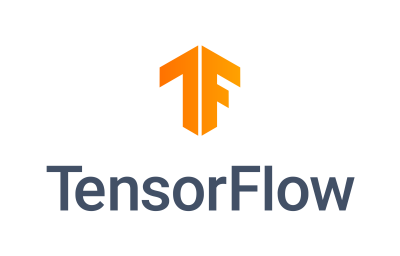Mostafa Shahverdy Husband, Father, Automating engineer, 🚀 SaaS Founder ☁️ AWS DevOps ❤️ Python 💻 AI / Machine Learning

# Introduction to TensorFlowTensorFlow is an open source platform for machine learning that provides a comprehensive and flexible ecosystem of tools, libraries and community resources. TensorFlow enables you to build, train, deploy and run machine learning models for various applications and domains.

As I feel confident enough on this topic, I feel the need to write about it. So, in this blog post, I will give you a brief overview of what TensorFlow is, how it works, and what you can do with it. Then later, I will try to continue writing on AI and Pythonic topics.

## What is TensorFlow?

TensorFlow is a software library for numerical computation using data flow graphs, where:

• Nodes in the graph represent mathematical operations.
• Edges in the graph represent the multidimensional data arrays (called tensors) communicated between them.

Tensors are the central unit of data in TensorFlow. They can have any shape and size, and can store any type of data, such as numbers, strings, images, etc. TensorFlow allows you to define and execute your computation graph in Python, C++, Java, Swift, or other languages. You can also use high-level APIs like Keras or TensorFlow Estimators to simplify the model building process. TensorFlow provides robust capabilities to deploy your models on any environment - servers, edge devices, browsers, mobile, microcontrollers, CPUs, GPUs, FPGAs. TensorFlow Serving can run ML models at production scale on the most advanced processors in the world, including Google’s custom Tensor Processing Units (TPUs).

## How does TensorFlow work?

TensorFlow works by following these steps:

• Define your computation graph using TensorFlow operations and tensors.
• Create a TensorFlow session to run your graph on a specific device (CPU, GPU, TPU, etc.).
• Feed your input data (such as images, text, audio, etc.) to the graph as tensors.
• Execute your graph and get the output tensors (such as predictions, classifications, embeddings, etc.).

For example, here is a simple TensorFlow program that adds two tensors:

``````1
2
3
4
5
6
7
8
9
10
11
12
13
14
15
16
17
import tensorflow as tf

# Define two tensors
a = tf.constant(2)
b = tf.constant(3)

# Define an operation that adds the tensors

# Create a session to run the graph
sess = tf.Session()

# Execute the graph and get the output tensor
result = sess.run(c)

# Print the result
print(result) # 5
``````

## What can you do with TensorFlow?

TensorFlow can help you solve various problems with machine learning, such as:

• Computer vision: You can use TensorFlow to build models that can recognize objects, faces, emotions, gestures, scenes, etc. from images or videos. For example, you can use TensorFlow to create a face detection app or a self-driving car system.
• Natural language processing: You can use TensorFlow to build models that can understand and generate natural language from text or speech. For example, you can use TensorFlow to create a chatbot or a speech recognition system.
• Generative models: You can use TensorFlow to build models that can generate realistic data from noise or latent variables. For example, you can use TensorFlow to create a style transfer app or a text summarization system.
• Reinforcement learning: You can use TensorFlow to build models that can learn from their own actions and rewards in an environment. For example, you can use TensorFlow to create a game-playing agent or a robot controller.
• And more: You can use TensorFlow to build models for any domain or application that involves data and learning from it. For example, you can use TensorFlow to create a recommendation system or a fraud detection system.